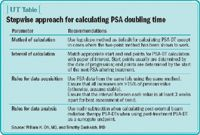# Practical rules for calculating PSA doubling time

Article

PSA doubling time, defined as the length of time it takes for a PSA to double based on an exponential growth pattern, and PSA velocity, the rate of change of PSA over time, have been identified in large retrospective studies as strong prognostic indicators at the time of diagnosis prior to prostatectomy in patients with prostate cancer.Both PSA-DT and PSA-V also have shown great promise in smaller retrospective studies as prognostic and predictive indicators at the time of development of androgen independence (J Urol 2004; 172:141-5; Cancer 2006; 106:63-7). Measurements of PSA kinetics have, therefore, become an essential part of urologists' knowledge base, assisting them in estimating prognosis once an initial diagnosis of prostate cancer has been established. Despite the growing base of literature proving the utility of PSA kinetics in predicting outcomes, there is surprisingly little consistency among studies regarding method of calculation. For PSA-DT, seemingly every study has different parameters for mathematical method, start and end points for calculation, and threshold PSAs for initiating calculation, minimum increases between values, and minimum time between values.Despite this, a stepwise approach can greatly simplify the process and ensure an appropriate estimate of PSA-DT. We recently evaluated the existing literature to identify the essential study-specific parameters for correct calculation of PSA-DT. These parameters, which are the focus of this article and which are summarized in the table, include method of calculation, interval of calculation, rules for data acquisition, and rules for data analysis.

Method of calculation

The calculation of PSA-DT is based on the fact that serum PSA levels in patients with newly diagnosed or recurrent prostate cancer follow an exponential growth curve, with the exponential rise of serum PSA beginning 7 to 9 years before the tumor is detected clinically (Cancer Res 1992; 52:3323-8). PSA rise can therefore be modeled by an exponential equation following first-order kinetics:

PSAt = PSAinitial * emt